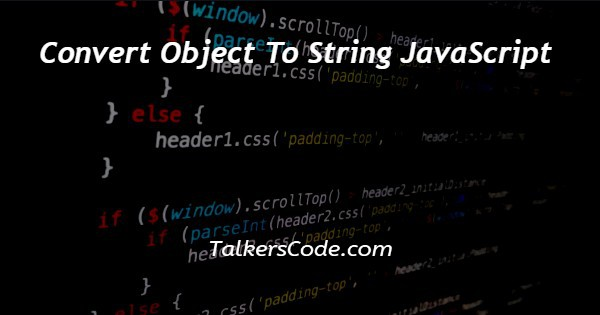# Convert Object To String JavaScript

Last Updated : Jan 1, 2023In this tutorial we will show you the solution of convert object to string JavaScript, there are many ways with help of which we can convert the object to a string using JavaScript.

So, let us see the ways with the help of the example given below.

## Step By Step Guide On Convert Object To String JavaScript :-

The ways with help of which we can convert the object to strings are:

• Using JSON.stringify() method
• Using string() function
• Using object.entries() method

Here in this article, we are going to understand our first way which is JSON.stringify().

Whereas remaining were discussed in our next tutorials. So, let us see the codes given below to understand about JSON.stringify() method.

```// convert object to string JavaScript

<script>
const array = ["Robert", "Thanos", "Steve", "Tom"];
const result = JSON.stringify(array);
console.log(result);
</script>
```
1. Here, above we show you an example in JavaScript.
2. We can also use basic HTML codes here to use this iteration inside HTML codes. We hope you all know what is the basic HTML structure and how to write this JavaScript code inside HTML tags.
3. If you don’t know anything from the above you can visit our article, in which you can learn these concepts easily from our experienced developers.
4. Now, when we heard about the object to string conversion. The first question that comes to mind that why we need to convert objects to strings. So, the answer is that to send the information or data between two different programming languages and two different platforms we have to convert the data into a form with help of which data can be used by both languages.
5. It means that there must a format with can be understood by all programming languages so that we can transfer data between different programming languages and platforms. So, to do this we convert our data into an object format which is received by another side. Now, due to some reason or another thing if we want to see the data that is transferred between platforms. Or make it understandable by the receiver side. We have to convert the data into the string format.
6. This is because the data in object format does not directly understandable. so, we need to convert it into the string format. So, for this today we use JSON.stringify() method. For example, according to the above example, we create an object or let us assume data is sent through a variable in object format. So, we have to convert the data from object format to string format to make this understandable. So, we use JSON.stringify() method and pass the variable as an argument to this function and store the result inside a variable. Now, after this, we just have to use that variable. For example, we just console the variable after stringify() to show the data after stringify() method.

## Conclusion :-

At last in conclusion, here we can say that with the help of this article we can understand how to add an object to an array using JavaScript.

I hope this tutorial on convert object to string JavaScript helps you and the steps and method mentioned above are easy to follow and implement.